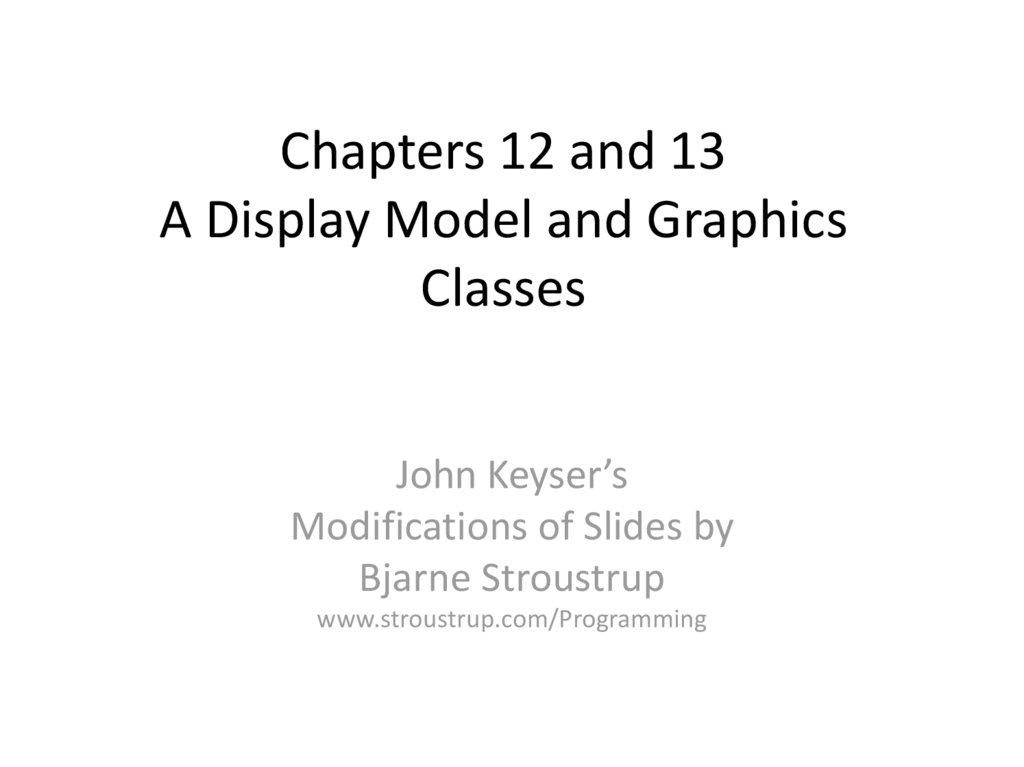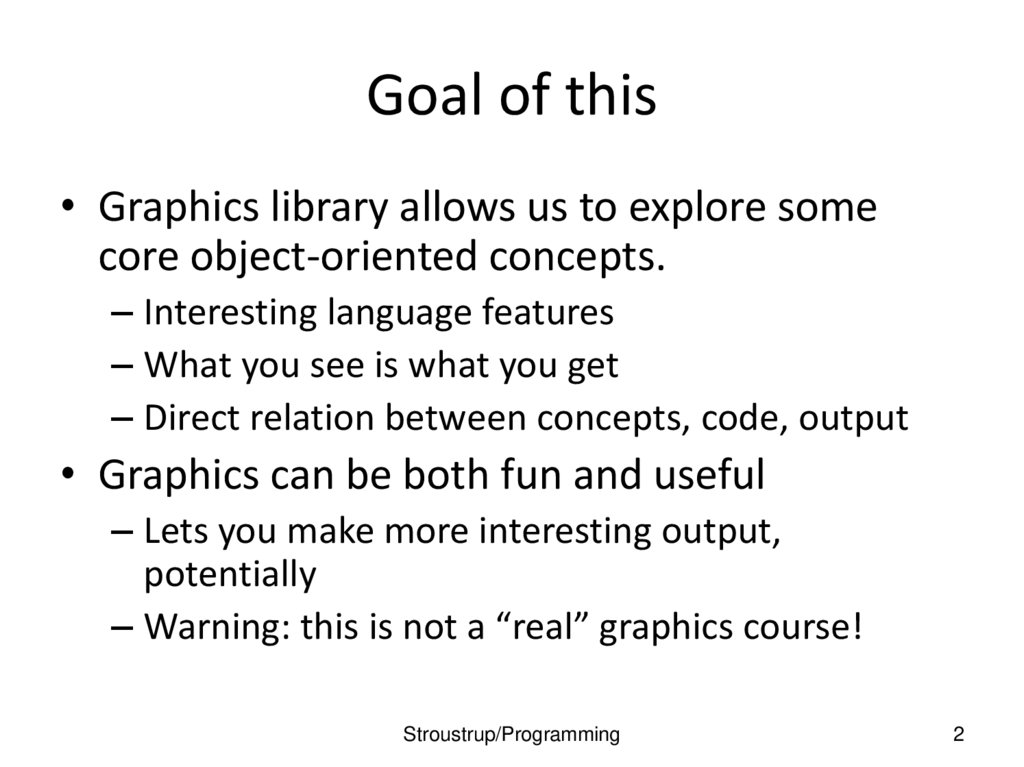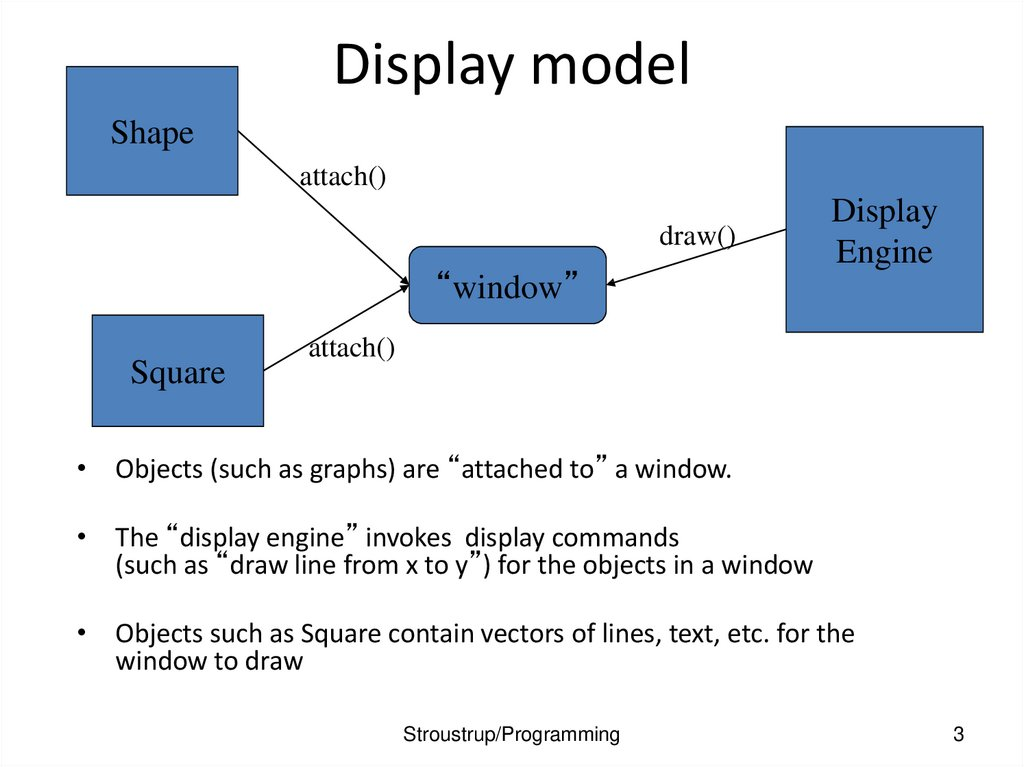# A Display Model and Graphics Classes

## 1. Chapters 12 and 13 A Display Model and Graphics Classes

John Keyser’s
Modifications of Slides by
Bjarne Stroustrup
www.stroustrup.com/Programming

## 2. Goal of this

• Graphics library allows us to explore some
core object-oriented concepts.
– Interesting language features
– What you see is what you get
– Direct relation between concepts, code, output
• Graphics can be both fun and useful
– Lets you make more interesting output,
potentially
– Warning: this is not a “real” graphics course!
Stroustrup/Programming
2

## 3. Display model

Shape
attach()
draw()
“window”
Square
Display
Engine
attach()
• Objects (such as graphs) are “attached to” a window.
• The “display engine” invokes display commands
(such as “draw line from x to y”) for the objects in a window
• Objects such as Square contain vectors of lines, text, etc. for the
window to draw
Stroustrup/Programming
3

## 4. Display model

• An example illustrating the display model
int main()
{
using namespace Graph_lib; // use our graphics interface library
Point tl(100,200);
// a point (obviously)
Simple_window win(tl,600,400,"Canvas");
// make a simple window
Polygon poly;
// make a shape (a polygon, obviously)
// add three points to the polygon
poly.set_color(Color::red);
// make the polygon red (obviously)
win.attach(poly);
// connect poly to the window
win.wait_for_button();
// give control to the display engine
}
Stroustrup/Programming
4

## 5. The resulting screen

Stroustrup/Programming
5

## 6. Graphics/GUI libraries

• You’ll be using a few interface classes we wrote
– Interfacing to a popular GUI toolkit
• GUI == Graphical User Interface
• FLTK: www.fltk.org
// Fast Light Tool Kit
– Installation, etc.
• See Appendix D and ask instructor/friend
– FLTK
– GUI and graphics classes developed for this course
– Project settings
• This model is far simpler than common toolkit interfaces
– The FLTK (very terse) documentation is 370 pages
– Our interface library is <20 classes and <500 lines of code
– You can write a lot of code with these classes
• And you can build more classes on them
Stroustrup/Programming
6

## 7. Graphics/GUI libraries (cont.)

• The code is portable
– Windows, Unix, Mac, etc.
• This model extends to most common graphics and GUI uses
• The general ideas can be used with any popular GUI toolkit
– Once you understand the graphics classes you can easily learn any
GUI/graphics library
• Well, relatively easily – these libraries are huge
Stroustrup/Programming
7

## 8. Graphics/GUI libraries

Our code
Our interface library
A graphics/GUI library (here FLTK)
The operating system (e.g. Windows or
Linux)
Our screen
• Often called “a layered architecture”
Stroustrup/Programming
8

## 9. Interface classes

Color
Window
Line_style
Shape
Point
Simple_window
Line
Lines
Polygon
… Rectangle
Text
• An arrow
means “is a kind of”
• Color, Line_style, and Point are “utility classes” used by the other classes
• Window is our interface to the GUI library (which is our interface to the screen)
Stroustrup/Programming
9

## 10. Interface classes

• Current

Color, Line_style, Font, Point,
Window, Simple_window
Shape, Text, Polygon, Line, Lines, Rectangle, …
Axis
• Easy to add (for some definition of “easy”)
– Grid, Block_chart, Pie_chart, etc.
• Later, GUI
– Button, In_box, Out_box, …
Stroustrup/Programming
10

## 11. Coordinates

Demo code 1
#include "Simple_window.h"
// stuff to deal with your system’s
windows
#include "Graph.h"
// graphical shapes
using namespace Graph_lib;
// make names available
// in main():
Simple_window win(Point(100,100),600,400,"Canvas");
// screen coordinate (100,100) is top left corner of window
// window size(600 pixels wide by 400 pixels high)
// title: Canvas
win.wait_for_button(); // Display!
Stroustrup/Programming
12

## 12. Demo code 1

A “blank canvas”
Stroustrup/Programming
13

## 13. A “blank canvas”

Demo code 2
Axis xa(Axis::x, Point(20,300), 280, 10, "x axis");
// make an Axis
// an axis is a kind of Shape
// Axis::x means horizontal
// starting at (20,300)
// 280 pixels long
// 10 “notches” (“tick marks”)
// text “x axis”
win.set_label("Canvas #2");
win.attach(xa);
// attach axis xa to the window
win.wait_for_button();
Stroustrup/Programming
14

## 14. Demo code 2

Stroustrup/Programming
15

Demo code 3
win.set_label("Canvas #3");
Axis ya(Axis::y, Point(20,300), 280, 10, "y axis");
ya.set_color(Color::cyan);
// choose a color for the axis
ya.label.set_color(Color::dark_red); // choose a color for the text
win.attach(ya);
win.wait_for_button();
Stroustrup/Programming
16

## 16. Demo code 3

Yes, it’s ugly, but this is a programming course, not a graphics design course
Stroustrup/Programming
17

## 17. Add a Y-axis (colored)

Demo code 4
win.set_label("Canvas #4");
Function sine(sin,0,100,Point(20,150),1000,50,50); // sine curve
// plot sin() in the range [0:100)
// with (0,0) at (20,150)
// using 1000 points
// scale x values *50, scale y values *50
win.attach(sine);
win.wait_for_button();
Stroustrup/Programming
18

## 18. Demo code 4

Stroustrup/Programming
19

## 19. Add a sine curve

Demo code 5
win.set_label("Canvas #5");
// I changed my mind about sine’s color
sine.set_color(Color::blue);
Polygon poly;
// make a polygon (a kind of Shape)
poly.add(Point(300,200)); // three points make a triangle
poly.set_color(Color::red);
poly.set_style(Line_style::dash);
// change the color
// change the line style
win.attach(poly);
win.wait_for_button();
Stroustrup/Programming
20

## 20. Demo code 5

Add a triangle (and color the curve)
Stroustrup/Programming
21

## 21. Add a triangle (and color the curve)

Demo code 6
win.set_label("Canvas #6");
Rectangle r(Point(200,200), 100, 50);
height
// top left point, width,
win.attach(r);
win.wait_for_button();
Stroustrup/Programming
22

## 22. Demo code 6

Stroustrup/Programming
23

Demo code 6.1
• Add a shape that looks like a rectangle
Closed_polyline poly_rect;
win.set_label("Canvas #6.1");
Stroustrup/Programming
24

## 24. Demo code 6.1

Add a shape that looks like a rectangle
But is it a rectangle?
Stroustrup/Programming
25

## 25. Add a shape that looks like a rectangle

Demo code 6.2
• We can add a point
// now poly_rect has 5 points
win.set_label("Canvas #6.2");
• “looking like” is not the same as “is”
Stroustrup/Programming
26

## 26. Demo code 6.2

Obviously a polygon
Stroustrup/Programming
27

## 27. Obviously a polygon

r.set_fill_color(Color::yellow); // color the inside of the rectangle
poly.set_style(Line_style(Line_style::dash,4));
// make the triangle fat
poly_rect.set_fill_color(Color::green);
poly_rect.set_style(Line_style(Line_style::dash,2));
win.set_label("Canvas #7");
Stroustrup/Programming
28

Stroustrup/Programming
29

Demo Code 8
Text t(Point(100,100),"Hello, graphical world!"); // add text
// point is lower left corner on the baseline
win.set_label("Canvas #8");
Stroustrup/Programming
30

## 30. Demo Code 8

Stroustrup/Programming
31

Demo Code 9
Modify text font and size
t.set_font(Font::times_bold);
t.set_font_size(20); // height in pixels
Stroustrup/Programming
32

## 32. Demo Code 9

Text font and size
Stroustrup/Programming
33

## 33. Text font and size

Image ii(Point(100,50),"image.jpg");
win.attach(ii);
win.set_label("Canvas #10");
// open an image file
Stroustrup/Programming
34

Stroustrup/Programming
35

Oops!
• The image obscures the other shapes
– Move it a bit out of the way
ii.move(100,200);
// move 100 pixels to the right (-100 moves left)
// move 200 pixels down (-200 moves up)
win.set_label("Canvas #11");
win.wait_for_button();
Stroustrup/Programming
36

## 36. Oops!

Move the image
Note how the parts of a shape that don’t fit in the window are “clipped” away
Stroustrup/Programming
37

## 37. Move the image

Demo Code 12
Circle c(Point(100,200),50);
Ellipse e(Point(100,200), 75,25);
e.set_color(Color::dark_red);
Mark m(Point(100,200),'x');
ostringstream oss;
oss << "screen size: " << x_max() << "*" << y_max()
<< "; window size: " << win.x_max() << "*" << win.y_max();
Text sizes(Point(100,20),oss.str());
Image cal(Point(225,225), "snow_cpp.gif"); // 320*240 pixel gif
// display center of image
win.set_label("Canvas #12");
win.wait_for_button();
Stroustrup/Programming
38

## 38. Demo Code 12

Stroustrup/Programming
39

## 39. Add shapes, more text

Boiler plate
#include "Graph.h"
#include “Simple_window.h"
interface
int main ()
try
{
// the main part of your code
}
catch(exception& e) {
cerr << "exception: " << e.what() << '\n';
return 1;
}
catch (...) {
cerr << "Some exception\n";
return 2;
}
Stroustrup/Programming
40

## 40. Boiler plate

Primitives and algorithms
• The demo shows the use of library primitives
– Just the primitives
– Just the use
• Typically what we display is the result of
– an algorithm
• Now we will look a bit more at how some of this is
implemented.
Stroustrup/Programming
41

## 41. Primitives and algorithms

Display model
Open_polyline
draw()
attach()
attach()
Rectangle
draw()
“window”
Display
Engine
draw()
• Objects (such as graphs) are “attached to” (“placed in”) a window.
• The “display engine” invokes display commands (such as “draw line
from x to y”) for the objects in a window
• Objects such as Rectangle add vectors of lines to the window to draw
Stroustrup/Programming
42

## 42. Display model

Code organization
Point.h:
struct Point { … };
FLTK code
GUI.h:
Window.h:
Graph.h:
// Graphing interface:
struct Shape { … };

// window interface:
class Window {…};

Graph.cpp:
// GUI interface:
struct In_box { … };

Window.cpp:
Graph code
Window code
chapter12.cpp:
#include "Graph.h"
#include "Window.h"
int main() { … }
Stroustrup/Programming
GUI.cpp:
GUI code
43

## 43. Code organization

Design note
• The ideal of program design is to represent concepts
directly in code
– We take this ideal very seriously
• For example:
– Window – a window as we see it on the screen
• Will look different on different operating systems (not our business)
– Line – a line as you see it in a window on the screen
– Point – a coordinate point
– Shape – what’s common to shapes
• (imperfectly explained for now; all details in Chapter 14)
– Color – as you see it on the screen
Stroustrup/Programming
45

## 44. Source files

Point
namespace Graph_lib
// our graphics interface is in Graph_lib
{
struct Point
// a Point is simply a pair of ints (the coordinates)
{
int x, y;
Point(int xx, int yy) : x(xx), y(yy) { }
};
// Note the ';'
}
Stroustrup/Programming
46

## 45. Design note

Line
struct Shape {
// hold lines represented as pairs of points
// knows how to display lines
};
struct Line : Shape
// a Line is a Shape defined by just two Points
{
Line(Point p1, Point p2);
};
Line::Line(Point p1, Point p2)
// construct a line from p1 to p2
{
// add p2 to this shape
}
Stroustrup/Programming
47

## 46. Point

Line example
// draw two lines:
using namespace Graph_lib;
Simple_window win(Point(100,100),600,400,"Canvas");
// make a window
Line horizontal(Point(100,100),Point(200,100));
// make a horizontal line
Line vertical(Point(150,50),Point(150,150)); // make a vertical line
win.attach(horizontal);
win.attach(vertical);
// attach the lines to the window
win.wait_for_button();
// Display!
Stroustrup/Programming
48

## 47. Line

example
Stroustrup/Programming
49

## 48. Line example

• Individual lines are independent
horizontal.set_color(Color::red);
vertical.set_color(Color::green);
Stroustrup/Programming
50

## 49. Line example

Lines
struct Lines : Shape {
// a Lines object is a set of lines
// We use Lines when we want to manipulate
// all the lines as one shape, e.g. move them all
// together with one move statement
void add(Point p1, Point p2); // add line from p1 to p2
void draw_lines() const;
// to be called by Window to draw Lines
};
• Terminology:

Lines “is derived from” Shape
Lines “inherits from” Shape
Lines “is a kind of” Shape
Shape “is the base” of Lines
• This is the key to what is called “object-oriented programming”
– We’ll get back to this in Chapter 14
Stroustrup/Programming
51

## 50. Line example

Lines Example
Lines x;
win.attach(x);
win.wait_for_button();
// horizontal line
// vertical line
// attach Lines object x to Window win
// Draw!
Stroustrup/Programming
52

## 51. Lines

example
• Looks exactly like the two Lines example
Stroustrup/Programming
53

## 52. Lines Example

Implementation: Lines
{
}
void Lines::draw_lines() const
// to somehow be called from Shape
{
for (int i=1; i<number_of_points(); i+=2)
fl_line(point(i-1).x, point(i-1).y, point(i).x, point(i).y);
}
• Note
– fl_line is a basic line drawing function from FLTK
– FLTK is used in the implementation, not in the interface to our classes
– We could replace FLTK with another graphics library
Stroustrup/Programming
54

## 53. Lines example

Draw Grid
(Why bother with Lines when we have Line?)
// A Lines object may hold many related lines
// Here we construct a grid:
int x_size = win.x_max();
int y_size = win.y_max();
int x_grid = 80;
// make cells 80 pixels wide
int y_grid = 40;
// make cells 40 pixels high
Lines grid;
for (int x=x_grid; x<x_size; x+=x_grid)
for (int y = y_grid; y<y_size; y+=y_grid)
// veritcal lines
// horizontal lines
win.attach(grid); // attach our grid to our window (note grid is one object)
Stroustrup/Programming
55

## 54. Implementation: Lines

Grid
Oops! Last column is narrow, there's a grid line on top of
the Next button, etc.—tweaking required (as usual)
Stroustrup/Programming
56

## 55. Draw Grid (Why bother with Lines when we have Line?)

Color
struct Color {
// Map FLTK colors and scope them;
// deal with visibility/transparency
enum Color_type { red=FL_RED, blue=FL_BLUE, /* … */ };
enum Transparency { invisible=0, visible=255 }; // also called Alpha
Color(Color_type cc) :c(Fl_Color(cc)), v(visible) { }
Color(int cc) :c(Fl_Color(cc)), v(visible) { }
Color(Color_type cc, Transparency t) :c(Fl_Color(cc)), v(t) { }
int as_int() const { return c; }
Transparency visibility() { return v; }
void set_visibility(Transparency t) { v = t; }
private:
Fl_Color c;
char v;
};
Stroustrup/Programming
57

## 56. Grid

Draw red grid
grid.set_color(Color::red);
Stroustrup/Programming
58

## 57. Color

Line_style
struct Line_style {
enum Line_style_type {
solid=FL_SOLID,
dash=FL_DASH,
dot=FL_DOT,
dashdot=FL_DASHDOT,
dashdotdot=FL_DASHDOTDOT,
};
// ------// - - - // .......
// - . - .
// -..-..
Line_style(Line_style_type ss) :s(ss), w(0) { }
Line_style(Line_style_type lst, int ww) :s(lst), w(ww) { }
Line_style(int ss) :s(ss), w(0) { }
int width() const { return w; }
int style() const { return s; }
private:
int s;
int w;
};
Stroustrup/Programming
59

## 58. Draw red grid

Example: colored fat dash grid
grid.set_style(Line_style(Line_style::dash,2));
Stroustrup/Programming
60

## 59. Line_style

Polylines
struct Open_polyline : Shape {
// open sequence of lines
};
struct Closed_polyline : Open_polyline {
// closed sequence of lines
void draw_lines() const
{
Open_polyline::draw_lines(); // draw lines (except the closing one)
// draw the closing line:
fl_line( point(number_of_points()-1).x,
point(number_of_points()-1).y,
point(0).x,
point(0).y
);
}
// not needed (why?)
};
Stroustrup/Programming
61

## 60. Example: colored fat dash grid

Open_polyline
Open_polyline opl;
Stroustrup/Programming
62

## 61. Polylines

Closed_polyline
Closed_polyline cpl;
Stroustrup/Programming
63

## 62. Open_polyline

Closed_polyline
• A Closed_polyline is not a polygon
– some closed_polylines look like polygons
– A Polygon is a Closed_polyline where no lines cross
• A Polygon has a stronger invariant than a Closed_polyline
Stroustrup/Programming
64

## 63. Closed_polyline

Text
struct Text : Shape {
Text(Point x, const string& s)
// x is the bottom left of the first letter
: lab(s),
fnt(fl_font()),
// default character font
fnt_sz(fl_size()) // default character size
// store x in the Shape part of the Text object
void draw_lines() const;
// … the usual “getter and setter” member functions …
private:
string lab;
// label
Font fnt;
// character font of label
int fnt_sz;
// size of characters in pixels
};
Stroustrup/Programming
65

## 64. Closed_polyline

Text t(Point(200,200), "A closed polyline that isn’t a polygon");
t.set_color(Color::blue);
Stroustrup/Programming
66

## 65. Text

Implementation: Text
void Text::draw_lines() const
{
fl_draw(lab.c_str(), point(0).x, point(0).y);
}
// fl_draw() is a basic text drawing function from FLTK
Stroustrup/Programming
67

Color matrix
• Let’s draw a color matrix
– To see some of the colors we have to work with
– To see how messy two-dimensional addressing can be
• See Chapter 24 for real matrices
– To see how to avoid inventing names for hundreds of objects
Stroustrup/Programming
68

## 67. Implementation: Text

Color Matrix (16*16)
Simple_window win20(pt,600,400,"16*16 color matrix");
Vector_ref<Rectangle> vr; // use like vector
// but imagine that it holds references to objects
for (int i = 0; i<16; ++i) {
// i is the horizontal coordinate
for (int j = 0; j<16; ++j) {
// j is the vertical coordinate
vr.push_back(new Rectangle(Point(i*20,j*20),20,20));
vr[vr.size()-1].set_fill_color(i*16+j);
win20.attach(vr[vr.size()-1]);
}
// new makes an object that you can give to a Vector_ref to hold
// Vector_ref is built using std::vector, but is not in the standard library
Stroustrup/Programming
69

## 68. Color matrix

(16*16)
More examples and graphics classes in the book (chapter 13)
Stroustrup/Programming
70

## 69. Color Matrix (16*16)

Next lecture
• What is class Shape?
• Introduction to object-oriented programming
Stroustrup/Programming
71